Next: Bibliography Up: -MDPs: Learning in Varying Previous: Stochastic Processes with Non-diminishing

# Event-learning with a background controller can be formulated as an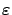-MDP

Lemma D.1 (Corollary 4.3)   Assume that the environment is such that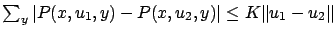for all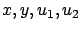. Letbe a prescribed number. For sufficiently large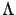and sufficiently small time steps, the SDS controller described in Equation 10 and the environment form an-MDP.

Proof. From [Szepesvári et al.(1997)] it is known that for sufficiently fine time steps, the eventual tracking error is bounded by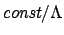, i.e., for sufficiently large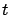,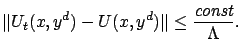For sufficiently large,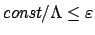. Therefore for arbitrary value function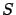we may write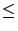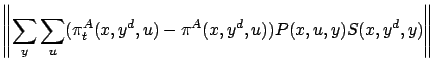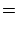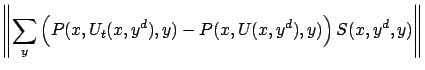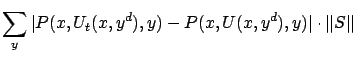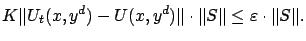This means that the system is indeed an-MDP.Naturally, if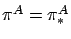then the approximated value function will be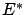.Next: Bibliography Up: -MDPs: Learning in Varying Previous: Stochastic Processes with Non-diminishing